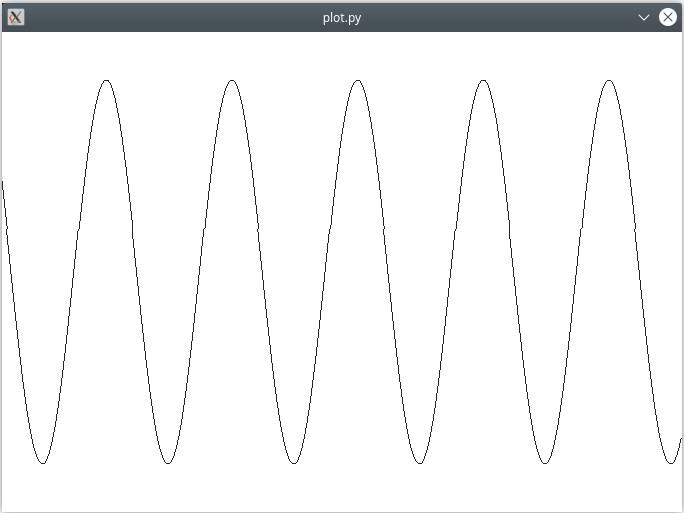# Plot Example#

The Plot example shows how to display a graph from data using an opaque container.

It draws an sine graph using `QPainter.drawPolyline()` from a list of points. The list of points is continuously updated, as is the case for a example for a graph of an oscilloscope or medical patient monitor. In this case, it makes sense from a performance point of view to avoid the conversion of a Python list of data to a C++ list (`QList<QPoint>`) for each call to the plot function `QPainter.drawPolyline()`. This is where opaque containers come into play.

Instead of Python list of points, a `QPointList` is instantiated to store the data. `QPointList` is an opaque container wrapping a `QList<QPoint>`. It can be passed to `QPainter.drawPolyline()` instead of a Python list of points.

The type is declared in the entry for the `QList` container type in the type system file of the `QtCore` library:

```<container-type name="QList" type="list"
opaque-containers="int:QIntList;QPoint:QPointList;QPointF:QPointFList">
...
</container-type>
```

In the `shift()` member function, new data are appended to the list while old data moving out of the visible window are removed from the front of the list.`Download this example`

```# Copyright (C) 2022 The Qt Company Ltd.

import math
import sys

from PySide6.QtWidgets import QWidget, QApplication
from PySide6.QtCore import QPoint, QRect, QTimer, Qt
from PySide6.QtGui import QPainter, QPointList

WIDTH = 680
HEIGHT = 480

class PlotWidget(QWidget):
"""Illustrates the use of opaque containers. QPointList
wraps a C++ QList<QPoint> directly, removing the need to convert
a Python list in each call to QPainter.drawPolyline()."""

def __init__(self, parent=None):
super().__init__(parent)
self._timer = QTimer(self)
self._timer.setInterval(20)
self._timer.timeout.connect(self.shift)

self._points = QPointList()
self._points.reserve(WIDTH)
self._x = 0
self._delta_x = 0.05
self._half_height = HEIGHT / 2
self._factor = 0.8 * self._half_height

for i in range(WIDTH):
self._points.append(QPoint(i, self.next_point()))

self.setFixedSize(WIDTH, HEIGHT)

self._timer.start()

def next_point(self):
result = self._half_height - self._factor * math.sin(self._x)
self._x += self._delta_x
return result

def shift(self):
last_x = self._points[WIDTH - 1].x()
self._points.pop_front()
self._points.append(QPoint(last_x + 1, self.next_point()))
self.update()

def paintEvent(self, event):
with QPainter(self) as painter:
rect = QRect(QPoint(0, 0), self.size())
painter.fillRect(rect, Qt.white)
painter.translate(-self._points.x(), 0)
painter.drawPolyline(self._points)

if __name__ == "__main__":

app = QApplication(sys.argv)

w = PlotWidget()
w.show()
sys.exit(app.exec())
```